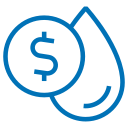Uh Oh! It seems you’re using an Ad blocker!

We always struggled to serve you with the best online calculations, thus, there's a humble request to either disable the AD blocker or go with premium plans to use the AD-Free version for calculators.

Or# Water Bill Calculator

Enter the total water amount and price per gallon to estimate water bill through this calculator.

Total Gallons of Water Used:

Price of Water ($/gallon): ADVERTISEMENT ADVERTISEMENT Table of Content Get the Widget! Add this calculator to your site and lets users to perform easy calculations. Feedback How easy was it to use our calculator? Did you face any problem, tell us! The water bill calculator calculates the total bill you need to pay for the water you have used in a single month. ## Working of Water Bill Calculator: Our water usage calculator gives you water bill estimates quickly! It requires a couple of inputs to estimate water bill that include: Inputs: • Enter the total amount of water in gallons • Likewise, enter the price per gallon of water • Tap Calculate Outputs: • Total water bill you need to pay • Steps involved Besides only using water for domestic purposes, you should also know your daily water drinking needs. This is why we have developed another water intake calculator that exactly calculates your daily water intake needs. ## How To Calculate Water Bills? If you want to estimate how much is the water bill for the consumption of water during the month, you may subject to the following formula: Water Bill ($) = Gallons of Water Used * Price of Water ($/Gallon) ### Example: Reshaeel used 600 gallons of water in a single month during which the water cost per gallon was$0.3. How to calculate water bill?

#### Solution:

Water Bill ($) = Gallons of Water Used * Price of Water ($/Gallon)

Water Bill ($) = 600 *$0.3

Water Bill ($) =$180

Except the water bill calculator, it may consume some precious minutes of your time. This is why using out water usage calculator will certainly give you water bill estimates in milliseconds.

## Average Water Bill Prices Per Month In 2023:

The following table highlights the most updated prices of water bills in the United States:

 National Average Cost $73 Typical Price Range$37-$105 Extreme Low-End Cost$20 Extreme High-End Cost \$115

## Faqs:

### How Is HCF Water Bill Calculated?

Basically, 1HCF is equivalent to 748 gallons of water. If you want to calculate HCF water bill, follow these steps:

• Hold your HCF water bill and note down the number of HCF
• Calculate the number by 748
• Divide the answer by the number of days for which the bill has been calculated

However, you may use our best water bill calculator to instantly estimate water bill invoice that is due.

### How Much Is 1 Kilolitre of Water?

1 kilolitre of water is equal to 1000 liters. If you consume this quantity of water in a month, then input it into the water bills calculator and it will instantly calculate the cost for the 1000 litres of water.

## References:

From the source Wikipedia: Water tariff, Tariff structures, Tariff levels, Tariff adjustment processes, Water supply, Technical overview